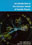© CAMBRIDGE UNIVERSITY PRESS 1998

1.2 The Construction of the Standard Model

Any theory of elementary particles must be consistent with special relativity. The combination of quantum mechanics, electromagnetism and special relativity led Dirac to the equation now universally known as the Dirac equation and, on quantising the fields, to quantum field theory. Quantum field theory had as its first triumph quantum electrodynamics, QED for short, which describes the interaction of the electron with the electromagnetic field. The success of a post-war generation of physicists, Feynman, Schwinger, Tomonaga, Dyson and others, in handling the infinities which arise in the theory led to a spectacular agreement between QED and experiment, which we describe in Chapter 8.

The Standard Model, like the QED it contains, is a theory of interacting fields. Our emphasis will be on the beauty and simplicity of the theory, and this can be understood at a certain ``classical'' level, treating the boson fields as true classical fields, and the fermion fields as completely anticommuting. To make a judgement of the success of the model in describing the data, it is necessary to quantise the fields, but to keep this book concise and accessible, results beyond the lowest orders of perturbation theory will only be quoted.

The construction of the Standard Model has been guided by principles of symmetry. The mathematics of symmetry is provided by group theory; groups of particular significance in the formulation of the Model are described in Appendix B. The connection between symmetries and physics is deep. Noether's theorem states, essentially, that for every continuous symmetry of Nature there is a corresponding conservation law. For example, it follows from the presumed homogeneity of space and time that the Lagrangian of a closed system is invariant under uniform translations of the system in space and in time. Such transformations are therefore symmetry operations on the system. It may be shown that they lead, respectively, to the laws of conservation of momentum and conservation of energy. Symmetries, and symmetry breaking, will play a large part in this book.

In the following sections of this chapter, we remind the reader of some of the salient discoveries of particle physics, which the Standard Model must incorporate. In Chapter 2 we begin on the mathematical formalism we shall need in the construction of the Standard Model.